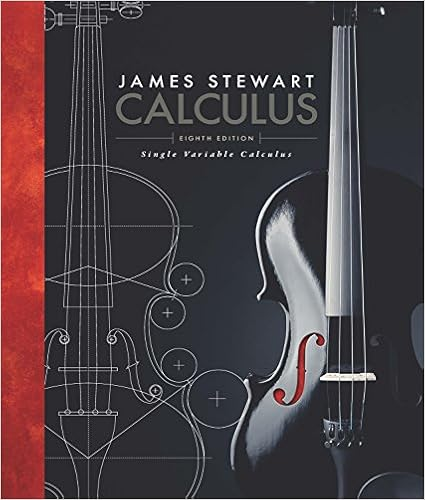# 77 to use the alternating series test consider a n f

• Homework Help
• rodblouin
• 66
• 100% (1) 1 out of 1 people found this document helpful

This preview shows page 33 - 35 out of 66 pages.

##### We have textbook solutions for you!
The document you are viewing contains questions related to this textbook.The document you are viewing contains questions related to this textbook.
Chapter 11 / Exercise 77
Single Variable Calculus
StewartExpert Verified
77. To use the alternating series test, consider a n = f ( n ) , where f ( x ) = arctan( a/x ) . We need to show that f ( x ) is decreasing. Since f 0 ( x ) = 1 1 + ( a/x ) 2 - a x 2 , we have f 0 ( x ) < 0 for a > 0 , so f ( x ) is decreasing for all x . Thus a n +1 < a n for all n , and as lim n →∞ arctan( a/n ) = 0 for all a , by the alternating series test, X n =1 ( - 1) n arctan( a/n ) converges. 78. The n th partial sum of the series is given by S n = 1 - 1 2 + 1 3 - · · · + ( - 1) n - 1 n , so the absolute value of the first term omitted is 1 / ( n + 1) . By Theorem 9.9, we know that the value, S , of the sum differs from S n by less than 1 / ( n +1) . Thus, we want to choose n large enough so that 1 / ( n +1) 0 . 01 . Solving this inequality for n yields n 99 , so we take 99 or more terms in our partial sum. 79. The n th partial sum of the series is given by S n = 1 - 2 3 + 4 9 - · · · + ( - 1) ( n - 1) 2 3 ( n - 1) ,
##### We have textbook solutions for you!
The document you are viewing contains questions related to this textbook.The document you are viewing contains questions related to this textbook.
Chapter 11 / Exercise 77
Single Variable Calculus
StewartExpert Verified
750 Chapter Nine /SOLUTIONS so the absolute value of the first term omitted is (2 / 3) n . By Theorem 9.9, we know that the value, S , of the sum differs from S n by less than (2 / 3) n . Thus, we want to choose n large enough so that (2 / 3) n 0 . 01 . Solving this inequality for n yields n 11 . 358 , so taking 12 or more terms in our partial sum is guaranteed to be within 0 . 01 of the sum of the series. Note: Since this is a geometric series, we know the exact sum to be 1 / (1+2 / 3) = 0 . 6 . The partial sum S 12 is 0 . 595 , which is indeed within 0 . 01 of the sum of the series. Note, however, that S 11 = 0 . 6069 , which is also within 0 . 01 of the exact sum of the series. Theorem 9.9 gives us a value of n for which S n is guaranteed to be within a small tolerance of the sum of an alternating series, but not necessarily the smallest such value. 80. The n th partial sum of the series is given by S n = 1 2 - 1 24 + 1 720 - · · · + ( - 1) n - 1 (2 n )! , so the absolute value of the first term omitted is 1 / (2 n + 2)! . By Theorem 9.9, we know that the value, S , of the sum differs from S n by less than 1 / (2 n +2)! . Thus, we want to choose n large enough so that 1 / (2 n +2)! 0 . 01 . Substituting n = 2 into the expression 1 / (2 n + 2)! yields 1 / 720 which is less than 0.01. We therefore take 2 or more terms in our partial sum. 81. Since 0 c n 2 - n for all n , and since 2 - n is a convergent geometric series, c n converges by the Comparison Test. Similarly, since 2 n a n , and since 2 n is a divergent geometric series, a n diverges by the Comparison Test. We do not have enough information to determine whether or not b n and d n converge. 82. (a) The sum a n · b n = 1 /n 5 , which converges, as a p -series with p = 5 , or by the integral test: Z 1 1 x 5 dx = lim b →∞ x - 4 ( - 4) b 1 = lim b →∞ b - 4 ( - 4) + 1 4 = 1 4 . Since this improper integral converges, a n · b n also converges. (b) This is an alternating series that satisfies the conditions of the alternating series test: the terms are decreasing and have limit 0 , so ( - 1) n / n converges. (c) We have a n b n = 1 /n , so a n b n is the harmonic series, which diverges.
•••